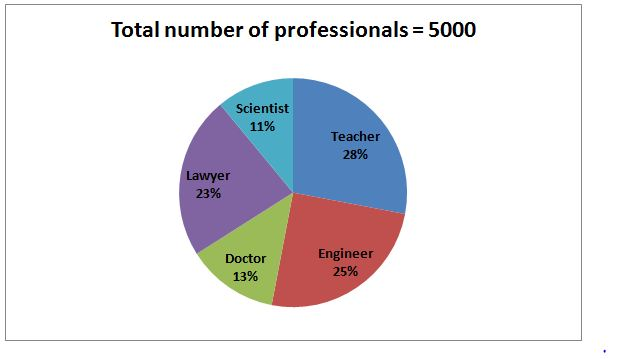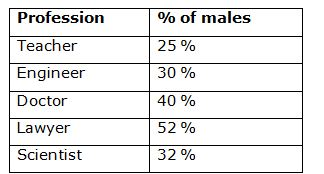# IBPS PO Prelims Quantitative Aptitude Questions 2019 (Day-12)

Dear Aspirants, Our IBPS Guide team is providing new series of Quantitative Aptitude Questions for IBPS PO Prelims 2019 so the aspirants can practice it on a daily basis. These questions are framed by our skilled experts after understanding your needs thoroughly. Aspirants can practice these new series questions daily to familiarize with the exact exam pattern and make your preparation effective.

Check here for IBPS PO Prelims Mock Test 2019

##### Check here for IBPS PO  Mock Test 2019
[WpProQuiz 7098]

Direction (1-5): What is the value should come in place of question mark in the following questions?

1) 16.67% of 36 + 33.33 % of 123*2 – √121 =?

a) 88

b) 77

c) 87

d) 65

e) 32

2) 34*36 + √529 * √121 – 93= ?

a) 788

b) 747

c) 748

d) 756

e) 810

3) ?/√384 = √864/?

a) 28

b) 19

c) 33

d) 24

e) 37

4) (6 x 136÷8 +132) / (628 ÷ 16 – 26.25) =?

a) 28

b) 18

c) 33

d) 24

e) 42

5) 42.85 % of 140 + 62.5 % of 120 – 16.67 % of 36 =?

a) 106

b) 118

c) 133

d) 142

e) 129

Directions (6 – 10): Study the following information carefully and answer the given questions.

The following pie chart shows the percentage distribution of total number of different professionals in a certain city.The table shows the percentage of male professionals among them.6) Total number of female teachers is approximately what percentage of total number of male doctors in a city?

a) 258 %

b) 312 %

c) 404 %

d) 450 %

e) 275 %

7) Find the ratio between the total number of male engineers to that of total number of female lawyers in a city?

a) 125: 184

b) 87: 134

c) 32: 59

d) 11: 23

e) None of these

8) Find the difference between the total number of teachers to that of scientist in a city?

a) 925

b) 775

c) 650

d) 850

e) None of these

9) Find the total number of female engineer, doctor and lawyer in a city?

a) 2589

b) 1817

c) 2345

d) 1692

e) None of these

10) Total number of female scientists is approximately what percentage of total number of male doctors in a city?

a) 58 % less

b) 36 % more

c) 44 % more

d) 44 % less

e) 58 % more

Directions (1-5):

?=1/6 of 36 + 1/3 of 123 *2 – 11 [16.67 % = 1/6, 33.33 % = 1/3]

?= 6+41*2-11

?= 6+82-11

?=77

? = (35 – 1) (35 + 1) + 23 *11 – 729 [a2 – b2 = (a + b) (a – b)]

? = 352 – 12 + 23 *11 – 729

? =1225 – 1+ 253 – 729

? =748

? * ? = √384 *√864

? * ? = √ (64x6x144x6)

? * ? =8x6x12 =576

? = 24

(6 x (136/8) + 132) / (628/16 – 26.25) =?

(102 + 132) / (39.25 – 26.25) = ?

234 / 13 =?

18 = ?

3/7 of 140 + 5/8 of 120 – 1/6 of 36 =?

[42.85 % = 3/7, 62.5 % = 5/8, 16.67 % = 1/6]

? = 60 + 75 – 6 = 129

Directions (6-10):

Total number of female teachers in a city

= > 5000*(28/100)*(75/100) = 1050

Total number of male doctors in a city

= > 5000*(13/100)*(40/100) = 260

Required % = [1050/260]*100 = 404 %

The total number of male engineers in a city

= > 5000*(25/100)*(30/100) = 375

The total number of female lawyers in a city

= > 5000*(23/100)*(48/100) = 552

Required ratio = 375: 552 = 125: 184

The total number of teachers in a city

= > 5000*(28/100) = 1400

The total number of scientist in a city

= > 5000*(11/100) = 550

Required difference = 1400 – 550 = 850

The total number of female engineer, doctor and lawyer in a city

= > [5000*(25/100)*(70/100)] + [5000*(13/100)*(60/100)] + [5000*(23/100)*(48/100)]

= > 875 + 390 + 552 = 1817

Total number of female scientists in a city

= > 5000*(11/100)*(68/100) = 374

Total number of male doctors in a city

= > 5000*(13/100)*(40/100) = 260

Required % = [(374 – 260)/260]*100 = 44 % more